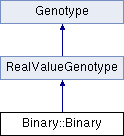# Binary::Binary Class Reference [Genotypes, Binary]

Binary class - implements genotype as a vector of binary coded real values with variable interval and precision. More...

`#include <Binary.h>`

Inheritance diagram for Binary::Binary:List of all members.

## Public Member Functions

uint getNumBits ()
Return number of bits used in a single dimension in real domain.
bool initialize (StateP state)
Initialize a genotype object (read parameters, perform sanity check, build data).
void registerParameters (StateP state)
Register genotype's parameters (called before Genotype::initialize).
bool update (void)
Update genotype after genetic material change via genetic operators.
Binarycopy ()
Create an identical copy of the genotype object.
std::vector< CrossoverOpP > getCrossoverOp ()
Create and return a vector of crossover operators.
std::vector< MutationOpP > getMutationOp ()
Create and return a vector of mutation operators.
void write (XMLNode &xBinary)
Write genotype data to XMLNode.
void read (XMLNode &xBinary)
Read genotype data from XMLNode.

## Public Attributes

std::vector< long int > decValue
integer values of bit representation
std::vector< v_bool > variables
vector of bit vectors

## Protected Types

typedef std::vector< bool > v_bool

## Protected Member Functions

double logbase (double a, double base)
double round (double, int)

## Protected Attributes

uint nDecimal_
number of digits after the decimal point
uint nBits_
number of bits for a single dimension
long potention_
bool bRounding_
use rounding with 'precision' digits on real values in the chromosome
v_bool vBool_
temporary bool vector

## Friends

class BinaryMutSimple

## Detailed Description

Binary class - implements genotype as a vector of binary coded real values with variable interval and precision.

Binary genotype parameters are (the first 3 are inherited from RealValueGenoptype):

• lbound: lower bound of represented real value
• ubound: upper bound of represented real value
• dimension: number of distinct real values, each coded with previous 3 parameters
• precision: desired number of digits (after the decimal point) to be represented
• rounding: whether the represented real value is rounded to 'precision' digits (false by default)

Definition at line 38 of file Binary.h.

The documentation for this class was generated from the following files: